PSAT Math : Equations / Solution Sets

Example Questions

1 2 3 5 Next →

Example Question #199 : Systems Of Equations

Give the solution to the system of equations below.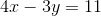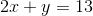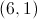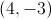No solution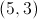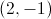Explanation:Solve the second equation for, allowing us to solve using the substitution method.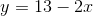Substitute forin the first equation, and solve for.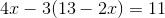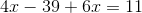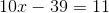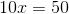Now, substitute forin either equation; we will choose the second. This allows us to solve for.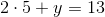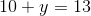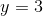Now we can write the solution in the notation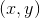, or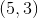.

Example Question #7 : Word Translations

Hannah is selling candles for a school fundraiser all fall. She sets a goal of selling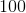candles per month. The number of candles she has remaining for the month can be expressed at the end of each week by the equations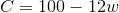, where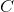is the number of candles and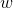is the number of weeks she has sold candles this month. What is the meaning of the value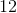in this equation?

The number of candles that she has sold thus far that week.

The number of candles that she sells each week.

The number of candles she has remaining for the month.

The number of weeks that she has sold candles this month.

Since we know thatstands for weeks, the answer has to have something to do with the weeks. This eliminates "the number of candles she has remaining for the month." Also, we can eliminate "the number of weeks that she has sold candles this month" because that would be our value for, not what we'd multiplyby. The correct answer is,  "the number of candles that she sells each week."